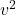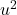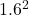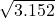A golfer needs to sink an 8m putt. She hits the ball, giving it an initial speed of 1.6 m/s, but it stops 1.5m short of the hole. c. What in

Question

A golfer needs to sink an 8m putt. She hits the ball, giving it an initial speed of 1.6 m/s, but it stops 1.5m short of the hole. c. What initial speed did the ball need to just make the putt? Assume the acceleration of the grass is constant. Hint: to just make the putt, the speed of the ball will be zero right at the hole. d. In order to make the 1.5m putt, what initial speed does she need to give the ball?

in progress 0
6 months 2021-07-29T13:51:51+00:00 1 Answers 8 views 0

1.78 m/s

Explanation:

Distance to putt = 8 m

Distance the ball stops to the putt = 1.5 m

therefore distance traveled by the ball = 8 – 1.5 = 6.5 m

The ball stops at this point 1.5 m from the putt, therefore its final velocity at this point = 0 m/s

the ball was struck with an initial velocity of 1.6 m/s

Using the equation=+ 2as

where v is the final velocity of the ball = 0 m/s

u is the initial speed of the ball = 1.6 m/s

a is the acceleration of the grass

s is the distance the ball travels = 6.5 m

substituting values, we have=+ 2(a x 6.5)

0 = 2.56 + 13a

13a = -2.56

a = -2.56/13 = -0.197 m/s^2

If this acceleration of the grass is assumed to be constant, then to the initial speed needed to make the putt will be calculated from=+ 2as

where

v is the final speed at the putt = 0 m/s

u is the initial speed with which the ball is struck = ?

a is the acceleration of the grass = -0.197 m/s^2

s is the distance to the putt = 8 m

substituting values, we have=+ 2(-0.197 x 8)

0 =– 3.152= 3.152

u == 1.78 m/s